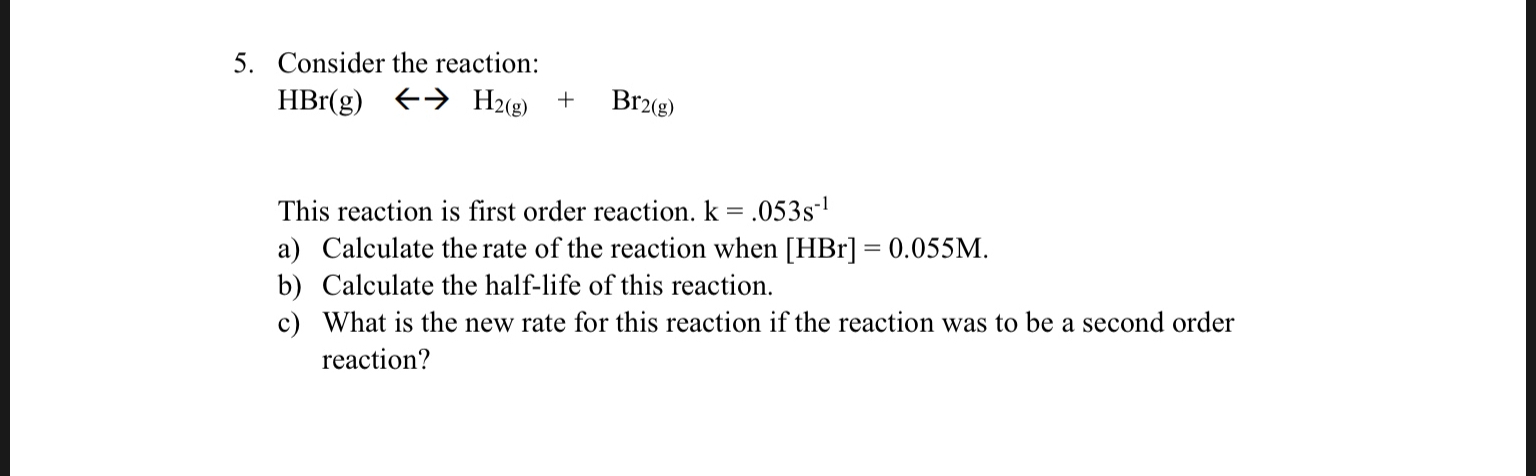# 5. Consider the reaction:H2(e)HBr(g)Br2(g)+This reaction is first order reaction. k = .053s1a) Calculate the rate of the reaction when [HBr] 0.055Mb) Calculate the half-life of this reaction.c) What is the new rate for this reaction if the reaction was to be a second orderreaction?

Question
30 viewshelp_outlineImage Transcriptionclose5. Consider the reaction: H2(e) HBr(g) Br2(g) + This reaction is first order reaction. k = .053s1 a) Calculate the rate of the reaction when [HBr] 0.055M b) Calculate the half-life of this reaction. c) What is the new rate for this reaction if the reaction was to be a second order reaction? fullscreen
check_circle

Step 1

The rate of the reaction for the first order reaction is written in equation (1) in which k is the rate constant of the reaction and A is the concentration of the reactant.

The half-life for a first order reaction is given by the equation (2) in which t1/2 is the half-life and k is the rate constant.

The rate of reaction for a second order reaction for a single reactant is shown in equation (3) in which k is the rate constant of the reaction and A is the concentration of the reactant.

Step 2

(a) The reaction is written as follows:

HBr (g) ⇌ H2(g) + N2(g)

Use the equation (1) to determine the rate of the above reaction and substitute 0.055M for A and 0.053 s-1for k as shown below.

Step 3

(b)

The half-life of the reaction is calculated by using the equation (...

### Want to see the full answer?

See Solution

#### Want to see this answer and more?

Solutions are written by subject experts who are available 24/7. Questions are typically answered within 1 hour.*

See Solution
*Response times may vary by subject and question.
Tagged in

### Chemistry